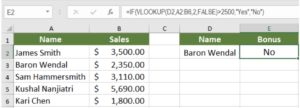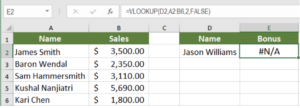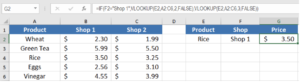Get instant live expert help with Excel or Google Sheets“My Excelchat expert helped me in less than 20 minutes, saving me what would have been 5 hours of work!”

#### Post your problem and you’ll get Expert help in seconds.

Your message must be at least 40 characters
Our professional Expert are available now. Your privacy is guaranteed.

# Learn How to Combine IF Function with VLOOKUP

VLOOKUP is a powerful function to perform lookup in Excel. It performs a row-wise lookup until a match is found. The IF function performs a logical test and returns one value for a TRUE result, and another for a FALSE result. IF and VLOOKUP functions are used together in multiple cases: to compare VLOOKUP results, to handle errors, to lookup based on two values. To use the IF and VLOOKUP functions together you should nest the VLOOKUP function inside the IF function.

## Combine IF Function with VLOOKUP

You can use IF and VLOOKUP together nesting the VLOOKUP function inside the IF function. The following will present a more detailed overview of the uses of IF function with VLOOKUP.

### Comparing values to VLOOKUP result

IF and VLOOKUP are used together mostly to compare the results of VLOOKUP to another value. In the following example, based on the list in cells A1:B6, to find out if the name mentioned in cell D2 has a bonus which is based on sales over \$2,500:

• Select cell E2 by clicking on it.
• Assign the formula `=IF(VLOOKUP(D2,A2:B6,2,FALSE)>2500,"Yes","No") `to cell E2.
• Press Enter to apply the formula in cell E2.This will return the result No as Baron Wendal had sales of \$2,350. You can also compare VLOOKUP results to cell values in a similar way. All you need to do is to set the cell reference as the condition inside the IF function.

### Handling errors

Another common use of IF function with VLOOKUP is to handle errors. In the previous example, assign the value Jason Williams to cell D2. To find the sales, assign the formula   = VLOOKUP(D2,A2:B6,2,FALSE) to cell E2.This will return a #N/A error. The name Jason Williams does not exist in cells A2:A6. To handle this error with your own custom message, you will nest the VLOOKUP and ISNA functions inside an IF function. To do that:

• Select cell E2 by clicking on it.
• Assign the formula =`IF(ISNA(VLOOKUP(D2,A2:B6,2,FALSE)),"Name not found",VLOOKUP(D2,A2:B6,2,FALSE)) `to cell E2.
• Press enter to apply the formula to cell E2.

This will return Name not found. The ISNA function checks if the result of the VLOOKUP is a #N/A error, and executes the corresponding IF condition.  You can set other text messages or even a 0 or blank (“”) as the output.

### Lookup Based on two values

You can also use IF and VLOOKUP together to perform a lookup based on two values. In this example, cells A1:C6 contains the price for products in two different shops. To find the price of the product in cell E2:

• Select cell G2 by clicking on it.
• Assign the formula `=IF(F2="Shop 1",VLOOKUP(E2,A2:C6,2,FALSE),VLOOKUP(E2,A2:C6,3,FALSE))` to cell G2.
• Apply the formula to G2 by pressing Enter.This will return \$3.50. The IF function checks if the value in cell F2 is Shop 1 or 2. According to this condition, the VLOOKUP then returns the corresponding price for the product.

Excel has several very effective functions when it comes to lookup values in other columns. One of these functions is VLOOKUP. It is a very powerful lookup and reference function for looking up data. Combined with the IF function, VLOOKUP can perform more advanced lookups that help to analyze data more effectively.

Still need some help with Excel formatting or have other questions about Excel? Connect with a live Excel expert here for some 1 on 1 help. Your first session is always free.

Are you still looking for help with the IF function? View our comprehensive round-up of IF function tutorials here.

How to Use a VLOOKUP in Excel – Excelchat

How to Use a VLOOKUP in Google Sheets – Excelchat

VLOOKUP Not Working? (Find Out Why) – Excelchat

How to Use VLOOKUP with Multiple Criteria – Excelchat

INDEX MATCH versus VLOOKUP: How and When to Use – Excelchat

How to Use VLOOKUP across Multiple Sheets in Excel – Excelchat

How to Use VLOOKUP with Multiple Workbooks – Excelchat

### Did this post not answer your question? Get a solution from connecting with the expert.Another blog reader asked this question today on Excelchat:## Subscribe to Excelchat.coAnother blog reader asked this question today on Excelchat: